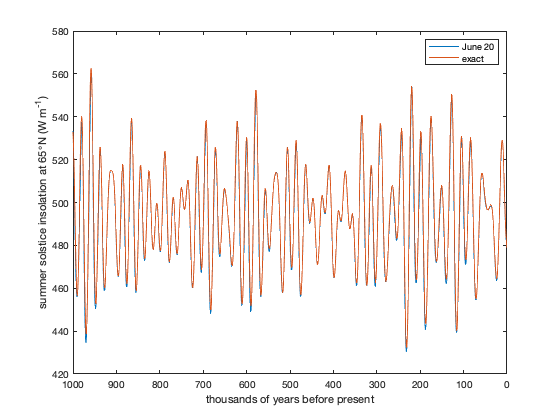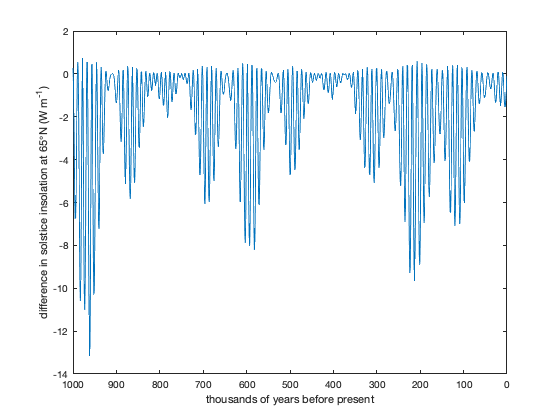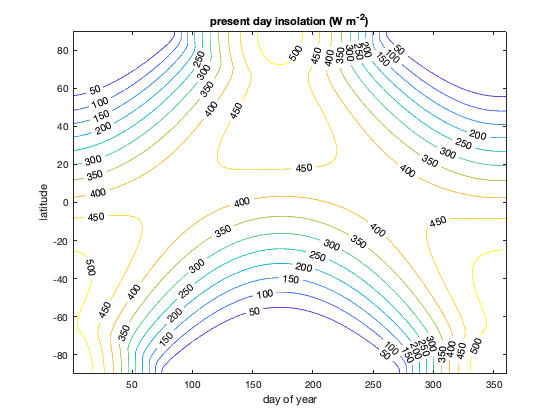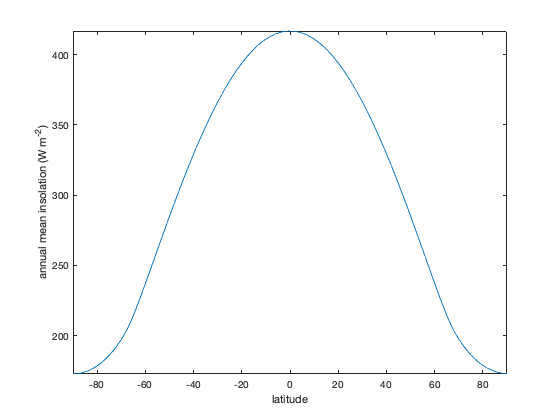# daily_insolation documentation

daily_insolation computes daily average insolation as a function of day and latitude at any point during the past 5 million years.

This function is from Ian Eisenman and Peter Huybers (see the reference below). The daily_insolation function is quite similar to the solar_radiation function, but one may suit your needs better than the other. The daily_insolation function is best suited for investigations involving orbital changes over thousands to millions of years, whereas the solar_radiation may be easier to use for applications such as present-day precipitation/drought research.

## Syntax

```Fsw = daily_insolation(kyear,lat,day)
Fsw = daily_insolation(kyear,lat,day,day_type)
Fsw = daily_insolation(kyear,lat,day,day_type,'constant',So)
Fsw = daily_insolation(kyear,lat,day,day_type,'mjmd')
[Fsw, ecc, obliquity, long_perh] = daily_insolation(...)```

## Description

Fsw = daily_insolation(kyear,lat,day) gives the daily average insolation in W/m^2 at latitude(s) on specified day(s) of the year (as given by the doy function) for the times kyear, which are thousands of years before present. For example, use kyear = +3000 to indicate 3 million years before present. Maximum allowed value of kyear is 5000.

Fsw = daily_insolation(kyear,lat,day,day_type) specifies an optional day type as 1 (default) or 2. The default option 1 specifies days in the range 1 to 365.24, where day 1 is January first and the vernal equinox always occurs on day 80. Option 2 specifies day input as solar longitude in the range 0 to 360 degrees. Solar longitude is the angle of the Earth's orbit measured from spring equinox (21 March). Note that calendar days and solar longitude are not linearly related because, by Kepler's Second Law, Earth's angular velocity varies according to its distance from the sun. If day_type is negative, kyear is taken to be a 3 element array containing [eccentricity, obliquity, and longitude of perihelion].

Fsw = daily_insolation(kyear,lat,day,day_type,'constant',So) specfies a solar constant So. Default So is 1365 W/m^2.

Fsw = daily_insolation(kyear,lat,day,day_type,'mjmd') returns Fsw in units of (MJ/m^2)/day rather than the default W/m^2.

[Fsw, ecc, obliquity, long_perh] = daily_insolation(...) also returns the orbital eccentricity, obliquity, and longitude of perihelion (precession angle).

## Example 1

For this example, calculate summer solstice insolation at 65 N. We'll do it two different ways. First, use doy to get the day of year corresponding to solstice, assuming it occurs on June 20:

```kyr = 0:1000;

Fsw_jun20 = daily_insolation(kyr,65,doy('june 20'));

plot(kyr,Fsw_jun20)
set(gca,'xdir','reverse') % reverses x direction
ylabel('summer solstice insolation at 65\circN (W m^{-1})')
xlabel 'thousands of years before present'
```Rather than approximating June 20 as the solstice, a more precise way would be to specify the exact orbital angle corresponding to the solstice (90 degrees) using the day_type=2 option, like this:

```Fsw_exact = daily_insolation(kyr,65,90,2);

hold on
plot(kyr,Fsw_exact)

legend('June 20','exact')
```## Example 2

We can plot the difference between June 20 (calendar day) and the exact summer solstice insolation at 65 N

```Fsw_jun20 = daily_insolation(kyr,65,doy('june 20'));
Fsw_exact = daily_insolation(kyr,65,90,2);

figure
plot(kyr,Fsw_jun20-Fsw_exact)
set(gca,'xdir','reverse') % reverses x direction
ylabel('difference in solstice insolation at 65\circN (W m^{-1})')
xlabel 'thousands of years before present'
```## Example 3

Insolation for the current orbital configuration as a function of day and latitude. Since this is a function of two variables, use meshgrid to create grids of the day and latitude variables:

```[day,lat]=meshgrid(1:5:365, -90:90);
[Fsw,ecc,obl,omega]=daily_insolation(0,lat,day);

disp([ecc,obl,omega]) % displays optional outputs
```
```    0.0172   23.4460  281.3700
```

We can now plot insolation as a function of day and latitude like this:

```figure
[c,h]=contour(day,lat,Fsw,[0:50:500]);
clabel(c,h)
xlabel 'day of year'
ylabel 'latitude'
title 'present day insolation (W m^{-2})'
```## Example 4

Calculate annual (not daily) average insolation by explicitly specifying orbital parameters.

```ecc=0.017236;
obl=23.446;
omega=101.37+180;
[day,lat]=meshgrid(1:5:365, -90:90);

Fsw=daily_insolation([ecc,obl,omega],lat,day,-1);

Fsw_annual = mean(Fsw,2);

figure
plot(-90:90,Fsw_annual)
axis tight
xlabel 'latitude'
ylabel 'annual mean insolation (W m^{-2})'
```## Example 5

Compare calculated insolation with example values given by Berger (1991). Start by loading Earth's orbital parameters from Berger A. and Loutre M.F., 1991, which is included as a sample dataset in CDT. Note, Berger and Loutre used negative values for their kiloyears, so we'll have to multiply them by -1. Also, they used a solar constant of 1360 W/m^2 so we'll specify that as well.

```D = importdata('orbit91.txt'); %

kyear = -D.data(:,1);
insol_65NJul = D.data(:,6);

Fsw=daily_insolation(kyear,65,(7-3)*30,2,'constant',1360);

figure
plot(kyear,1-insol_65NJul./Fsw)
```Note, the values above agree within 3e-5.

## Example 6

Plot 65N integrated summer insolation as in Huybers (2006), Science 313 508-511:

```[kyear,day]=meshgrid(1000:1:2000,1:1:365);
Fsw = daily_insolation(kyear,65,day);
Fsw(Fsw<275)=0;

figure
plot(-(1000:1:2000),sum(Fsw,1)*86400*10^-9)
title('As in Huybers (2006) Fig. 2C')
```## Detailed description of calculation:

Values for eccentricity, obliquity, and longitude of perihelion for the past 5 Myr are taken from Berger and Loutre 1991 (data from ncdc.noaa.gov). If using calendar days, solar longitude is found using an approximate solution to the differential equation representing conservation of angular momentum (Kepler's Second Law). Given the orbital parameters and solar longitude, daily average insolation is calculated exactly following Berger 1978.

## References:

• Berger A. and Loutre M.F. (1991). Insolation values for the climate of the last 10 million years. Quaternary Science Reviews, 10(4), 297-317.
• Berger A. (1978). Long-term variations of daily insolation and Quaternary climatic changes. Journal of Atmospheric Science, 35(12), 2362-2367.
• Huybers, Peter. "Early Pleistocene glacial cycles and the integrated summer insolation forcing." science 313.5786 (2006): 508-511.

## Authors:

Ian Eisenman and Peter Huybers, Harvard University, August 2006 eisenman@fas.harvard.edu. Slightly modified by Chad A. Greene for inclusion in the Climate Data Toolbox for Matlab, 2019.